Bicycle Stability

Introduction

Our current understanding of steering dynamics and self-stability of modern bicycles is not complete, due to the complexity of the mechanical analysis. Consequently, it is hard to get concrete design guidelines to achieve or prevent particular behaviors of the bicycle. However, we can start by studying the governing equations for a reasonably complex idealized bicycle, and develop more realistic models from there.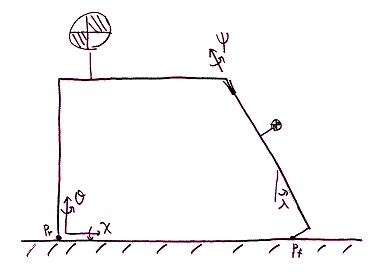Fig. 1: Skeleton Frame; χ is the lean to the right of the rear assembly (rotation about horizontal axis); θ is the heading of the rear aseembly (rotation about a vertical axis); ψ is the angle of steer of the front assembly relative to the rear assembly.

Bicycle Equations of Motion

In deriving the equations of motion, we basically followed the arguments by Richard Scott Hand  and by Jim M. Papadopoulos . The relevant quantities are defined in Fig. 1 and Fig. 2. The ideal bicycle we are studying can be considered as two bodies - a rigid rear-frame with rigidly attached immobile rider and a rigid steerable front-frame (fork + stem +bars) - joined at the steering axis. The torques for rotational accelerations d2χ/dt2, d2θ/dt2, d2ψ/dt2 are obtained from the moment of inertia tensors of the front and rear assemblies, assuming that the wheels are not rotating. The actual rotations of wheels are incorporated by introducing a quantity of angular momentum Hr attached to rear assembly, and a quantity Hf attached to front assembly. Aerodynamic forces, bearing resistance, and rolling resistance are neglected , .

As shown in Fig. 2, the configuration of the bicycle and the positions of mass points on it are determined by Y, X, θ, χ, ψ, and the amount of rotation of both wheels. In our model, the bicycle has small deviations from upright coasting along the Y axis of a level plane where the forward velocity V is essentially constant. Therefore, Y = Vt and each wheel rotates at a nearly constant rate. We consider V, Hr, and Hf as constants and the variables X, θ, χ, ψ as very small .

Under these assumptions, we get four equations of motion, each involving the total X-force, the total χ-moment, the total θ-moment, and the total ψ-moment respectively. Considering the particular case where the wheels have zero side slips, we then obtain two reduced equations:

Mχχd2χ/dt2 + Mχψd2ψ/dt2 + Cχψdψ/dt + Kχχχ + Kχψψ = 0 (lean equation)
Mψχd2χ/dt2 + Mψψd2ψ/dt2 + Cψχdχ/dt + Cψψdψ/dt + Kψχχ + Kψψψ = Mψ (steer equation)

The M, C, and K coefficients are determined by a given bicycle configuration, and defined in Papadopoulos's paper . They are all constants at a given bicycle velocity V.

Bicycle Self-Stability

Self-stability denotes the phenomenon that the bicycle returns to an upright configuration after any moderate disturbance. For no-hands motion of a bicycle, we eliminate one variable from the lean equation and steer equation, and get the following equation in terms of differential operators:

(Mχχd2/dt2 + Kχχ) (Mψψd2/dt2 + Cψψd/dt + Kψψ) - (Mψχd2/dt2 + Cψχd/dt + Kψχ)(Mχψd2/dt2 + Cχψd/dt + Kχψ) = 0, or
Ad4/dt4 + Bd3/dt3 + Cd2/dt2 + Dd/dt + E = 0

Here, A = a0, B = bV, C = co+c2V2, D = d1V+d3V3, E=e0+e2V2 for some parameters a0, ..., e2 determined by the coefficients M, C, and K.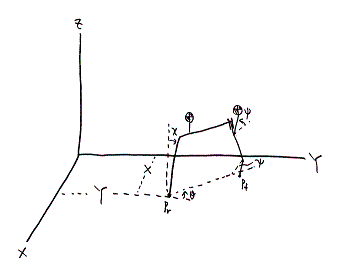Fig. 2: Picture of skeleton frame in general configuration; X is displacement of Pr from Y-axis

It is hard to solve the above equation of a given bicycle using a computer, since it requires a tremendous number of computer runs. Also, solving the equation analytically is extremely difficult. In fact, whether leaning and steering motions eventually damp out or not is determined by the signs of the real parts of the equation's eigenvalues. Thus, one reasonable approach would be to apply Routh-Hurwitz tests:

A, B, C, D, E > 0
C - A(D/B) - E(B/D) = RH > 0

If a bicycle satisfies the above criteria, then it is stable.

In a conventional design, the parameters a0, b1, c2, d3, e0 in the above equation are positive, and c0, d1, e2 are negative. Therefore, the velocity needs to be sufficiently large to make C, D, and RH positive, but it should not be too great for E to be positive. In general, bicycle achieves self-stability in a single range of speeds if at all (12 mph - 16 mph for a usual bicycle), and the limiting speeds occur when RH = 0 and E = 0.

RH = 0 is the condition for the existence of purely imaginary eigenvalues. In this case, V is typically the low-speed limit, and the bicycle executes a weaving motion which does not damp away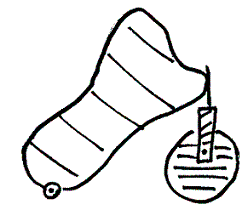Fig. 3a: Simple Primitive Bicycle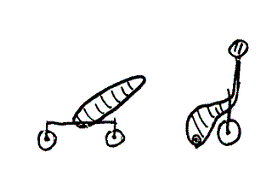Fig. 3b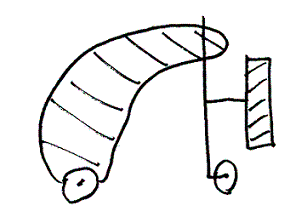Fig. 3c

E = 0 is the condition for a zero eigenvalue, where the corresponding velocity is usually the high-speed limit. At a slightly faster speed, the bicycle tends to fall over in any turn without any weaving. -E is proportional to the steering moment applied by the rider when the bicycle is balanced in any turn. Thus, the handlebars should be restrained from turning further for a stable bicycle.

Special Cases in Stability Analysis

Besides making general observations from Routh-Hurwitz tests, we can study simple bicycle designs to determine whether they satisfy the tests.

(1) If the gyroscopic effects of the front and rear wheels are somehow canceled, then the quantity d3 becomes zero so D has the same sign with that of d1. For a bicycle with standard dimensions and mass-distribution, d1 is generally negative. Therefore, a bicycle cannot achieve stability in this case.

(2) Fig. 3(a) illustrates a "primitive" bicycle having a vertical steering axis, front assembly symmetrical about the steering axis, and arbitrary distribution of mass on the rear assembly. For this design, E = 0 and RH < 0, so it is unstable. If the rear assembly has enough high-up mass in front of the steering axis as in Fig. 3(b), RH can be made positive so weaving instability is resolved. Yet, E is not positive so the bicycle does not straiten up from a turn. By making a positive trail or by moving the front-assembly center of mass ahead of the steering axis (Fig. 3(c)), a primitive bicycle can be stabilized.

Conclusion

Analyzing behaviors of a bicycle is complicated even with simplified equations of motion. However, self-stability of a given bicycle can be determined rather easily by applying Routh-Hurwitz tests to its configuration. The tests can be used to various simplified models of a bicycle, and provide useful design guidelines.

© 2007 Yong Suk Moon. The author grants permission to copy, distribute and display this work in unaltered form, with attribution to the author, for noncommercial purposes only. All other rights, including commercial rights, are reserved to the author.

References

 R. S. Hand, "Comparison and Stability Analysis of Linearized Equations of Motion for a Basic Bicycle Model," ScM. Thesis, May 1988.

 J. M. Papadopoulos, "Bicycle Steering Dynamics and Self-Stability: A Summary Report on Work in Progress," Cornell Bicycle Research Project, December 1987.

 J. Olsen and J. M. Papadopoulos, "Bicycle Dymanics - The Meaning Behind the Math," Bike Tech, vol. 7, issue 6, pages 13-15, Dec 1988.

 S. Goyal, "Second Order Kinematic Constraint between Two Bodies Rolling, Twisting and Slipping against each other While Maintaining Point Contact," Cornell technical report TR 89-1043, October 1989.